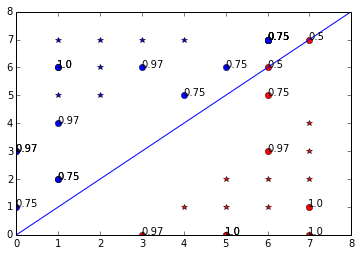# Logistic Regression using Theano¶

In :
# Import the required modules
%pylab inline
from theano import tensor as T
from theano import function
from theano import pp
from theano import Param
from theano import shared
import numpy as np

class LogisticRegression:
'''
This class performs logistic regression.
'''
def __init__(self, alpha=0.001, iterations=10000, threshold=.5):
'''
1. alpha is the learning rate.
The default value of alpha is 0.001.
2. iterations is the number of times the weights will be updated.
The default value is 1000
3. threshold is the threshold used to convert the probability
to either 0 or 1 class label
'''
# Set the learning rate
self.alpha = alpha
# Set the number of iterations
self.iterations = iterations
# Set the threshold value
self.threshold = threshold

# Define the symbolic Variables
X, W = T.dmatrices('training-set', 'weights')
y = T.matrix('testing-set')
m, a = T.scalars('no-of-samples', 'learning-rate')

# Define the cost function and the gradient functions
sigmoid_fn = 1/(1 + T.exp(-T.dot(X, W)))
h = sigmoid_fn
cost_fn = -((T.dot(y, T.transpose(h)) + T.dot(1 - y, T.transpose(1 - h)))/(1 * m)).sum()
grad_fn = (T.dot(T.transpose(X), h - y))/(m)
updated_wts = W - a * grad_fn

# Compile the Theano functions
self.cost = function([X, y, W, m], cost_fn)
self.new_wts = function([X, y, W, m, a], updated_wts)
self.pred = function([X, W], h)

def fit(self, X, y):
'''
The fit member function is used to train the classifier.
1. X is array-like data vector
2. y is array-like target vector
'''
# Add the bias feature to the data samples
bias = np.ones((X.shape, 1))
X = np.hstack((X, bias))

# m is the number of data samples used
m = X.shape

# Initialize all weights to 0
self.W = np.zeros((X.shape,1), dtype=np.float)

# Iterate and update the cost function and the weights
for i in xrange(self.iterations):
cst = self.cost(X, y, self.W, m)
self.W = self.new_wts(X, y, self.W, m, self.alpha)

# Set the coef_ and intercept_
self.coef_ = self.W[:-1]
self.intercept_ = self.W[-1]

def predict(self, X):
'''
This is function to predict target value.

1. X is array-like data vector whose target vector is predicted
'''
# Add the bias feature to the data samples
bias = np.ones((X.shape, 1))
X = np.hstack((X, bias))

# Return the predicted target label
return (self.pred(X, self.W) > self.threshold) * 1

def predict_proba(self, X):
'''
This is function gives the probability of how sure.

1. X is array-like data vector whose target vector is predicted
'''
# Add the bias feature to the data samples
bias = np.ones((X.shape, 1))
X = np.hstack((X, bias))

# Return the predicted target label
prob = self.pred(X, self.W)
for index, value in enumerate(prob < self.threshold):
if value:
prob[index] = 1 - prob[index]
return prob

Populating the interactive namespace from numpy and matplotlib

In :
# Prepare the train data
x1 = [[x, y] for x in range(3, 8) for y in range(1, 6) if x - 3 > y - 1 ]
x2 = [[x, y] for x in range(1, 6) for y in range(3, 8) if y - 3 > x - 1 ]
X_train = []
for x in x1:
X_train.append(x)
for x in x2:
X_train.append(x)
X_train = np.array(X_train)

# Prepare the train data labels
y1 = []*len(x1)
y2 = []*len(x2)
y_train = []
for y in y1:
y_train.append(y)
for y in y2:
y_train.append(y)
y_train = np.array(y_train)

# Prepare the test data
X_test = [[randint(0, 8) , randint(0, 8)] for x in range(25)]
X_test = np.array(X_test)

# Fit and predict
clf = LogisticRegression(iterations=10000)
clf.fit(X_train, y_train)
y_pred = clf.predict(X_test)
y_pred_prob = clf.predict_proba(X_test)

In :
# NOTE :: This graphic will only work for 2-D features, although the
# code can work for any dimensions
# Plot the boundary
# Training set in stars
# Testing set in circles
# Also show the probability
plot(X_train[:,0][y_train[:,0]==0], X_train[:,1][y_train[:,0]==0], "*r")
plot(X_train[:,0][y_train[:,0]==1], X_train[:,1][y_train[:,0]==1], "*b")
plot(X_test[:,0][y_pred[:,0]==0], X_test[:,1][y_pred[:,0]==0], "or")
plot(X_test[:,0][y_pred[:,0]==1], X_test[:,1][y_pred[:,0]==1], "ob")
x_min, x_max, y_min, y_max = 0 , 8, 0, 8
axis([x_min, x_max, y_min, y_max])
y_min_val = (clf.coef_ * x_min + clf.intercept_)/-clf.coef_
y_max_val = (clf.coef_ * x_max + clf.intercept_)/-clf.coef_
plot([x_min, x_max], [y_min_val, y_max_val])
for index, (x, y) in enumerate(X_test):
text(x, y, round(y_pred_prob[index], 2))